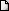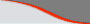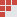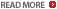# IIM CAT sample questions : Quadratic equations - 2nd order polynomials - Algebra : Ascent Education - MBA coaching coursesLATEST NEWS • Ascent Success Stories • TANCET 16 Classes @ Chennai • XAT 16 Classes @ ChennaiHome | TANCET Coaching Class | TANCET study material | Online TANCET Course | Contact us
 Ascent's Courses TANCET Coaching Chennai Online TANCET Course TANCET MBA Study Material CAT Classroom Chennai CAT Correspondence India Mock CAT Series GRE Coaching Chennai GMAT Coaching Chennai Online GMAT CourseUS B Schools Faculty List Jobs @ Ascent Testimonials Ascent Success Stories Contact Us +91 44 4500 8484 +91 96000 48484
You are here: Home » CAT Math » Algebra » Quadratic equations - roots

#### Quadratic Equations Problems : CAT 2016 Quant / Math Prep

Question 4 the day : March 12, 2003
The question for the day is from the topic Quadratic Equations. The question is about finding the value of an expression after evaluating the roots of a quadratic equation.

Question
If p and q are the roots of the equation x2 - bx + c = 0, then what is the equation if the roots are (pq + p + q) and (pq - p - q)?

(1) x2 - 2cx + (c2 - b2) = 0
(2) x2 - 2bx + (b2 + c2) = 0
(3) Bcx2 - 2(b+c)x + c2 = 0
(4) x2 + 2bx - (c2 - b2) = 0

Correct Choice is (1). Correct Answer is x2 - 2cx + (c2 - b2) = 0

In the given quadratic equation x2 - bx + c = 0,
The sum of the roots p + q = b --- (1)
And the product of the roots pq = c --- (2)

We have to formulate a quadratic equation whose roots are (pq + p + q) and (pq - p - q).

The sum of the two roots = pq + p +q + pq - p - q = 2pq
But from eqn (2), we know that pq = c
Therefore, the sum of the roots = 2c

The product of the roots = (pq + p + q)(pq - p - q)= (pq)2 - (p+q)2
From equation (1) and (2), we know that pq = c and p + q = b
Therefore, the product of the roots = c2 - b2

We know the sum of the roots and the product of the roots.
Therefore, the quadratic equation is x2 - (sum of the roots)x + product of the roots = 0

=> x2 - 2cx + c2 - b2 = 0

## More Questions on Linear, Quadratic and Cubic Equations - Algebra

 5. 6. 7.

## XLRI XAT TANCET Practice Question Samples

TANCET Questionbank
 TANCET MBA MCA Practice Questions with detailed explanation and shortcuts.GMAT Math Books
 Comprehensive study material for GMAT Quant. 1800+ questions.Staying outside India?
 Ascent offers CAT Correspondence Course for those who reside abroad.TANCET Diagnostic Test

CAT classes @ Chennai
 CAT 2010 intensive batch starts Jan 23, 10. Faculty includes IIM graduates. Personal attention. Online Mock CATs & Analysis.GMAT Math Lesson Book | SAT prep | GRE Classes @ Chennai | GMAT Coaching @ Chennai | TANCET Mailing List © 2002 - 17 ASCENT Education all rights reserved. Terms & Condition | Privacy Policy